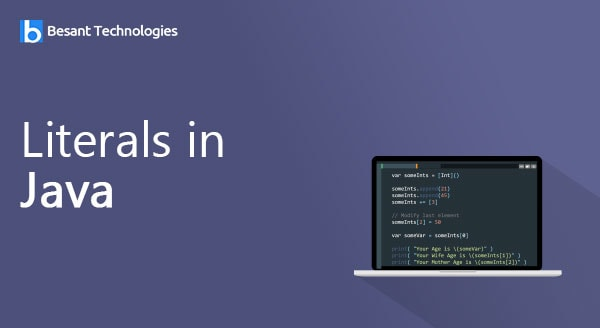Our Special Offer - Get 3 Courses at 24,999/- Only. Read more
Hire Talent (HR):+91-9707 240 250

# General# Literals in Java

## Literals in Java

Literals is an identifier whose value is fixed and does not change during the execution of the program.

### Integer Literals

Integer Literals are numbers that has no fractional pars or exponent. It refers to the whole numbers. Integers always begin with a digit or + or -.

We can specify integer constants in

• Decimal
• Octal

#### Decimal Integer Literals

It consists of any combination of digits taken from the set 0 to 9.

##### Example:
```int a = 100;   //Decimal Constant
int b = -145   // A negative decimal constant
int c = 065    // Leading zero specifies octal constant, not decimal```

#### Octal Integer Literals

It consists of any combination of digits taken from the set 0 to 7. However the first digit must be 0, in order to identify the constant as octal number.

Example:

```int a = 0374;  //Octal Constant
int b = 097;  // Error: 9 is not an octal digit.

```

A Sequence of digits begin the specification with 0X or 0X, shadowed by a system of digits in the range 0 to 9 and A (a) to F(f).

Example:

```int  a = 0x34;
int b = -0XABF;

```

### Unsigned Literals

We use either u or U suffix for Unsigned Constants and use either the l or L suffix. for Long constants

Example :

```328u  0x7FFFFFL  0776745ul;

```

### Floating-point Literals

Floating-point Literals are also called as real constants. The Floating Point contains decimal points and can contain exponents. They are used to represent values that will have a fractional part and can be represented in two forms – fractional form and exponent form.

In the fractional form, the fractional number contains the integer part and fractional part. A dot (.) is used to separate the integer part and fractional part.

Example:

`float x = 2.7f;`

In the exponential form, the fractional number contains constants a mantissa and exponent. Mantissa contains the value of the number and the exponent contains the magnitude of the number. The exponent, if any present, specifies the magnitude of the number as a power of 10.

Example:

``` 7.6: 23.46e0 // 23.46 x 100 = 23.46 x 1 = 23.46 23.46e1 // 23.46 x 101= 23.46 x10 = 234.6

```

### Character Literals

Character Literals are specified as single character enclosed in pair of single quotation marks. Single character constants are internally represented as ASCII codes.

### String Literals

String Literals are treated as an array of char. By default, the compiler adds a special character called the ‘null character’ (‘\0’) at the end of the string to mark the end of the string.

Example:

```String str = “good morning”;

```

### Boolean literals

There are two Boolean literals

• true represents a true Boolean value
• false represents a false Boolean value